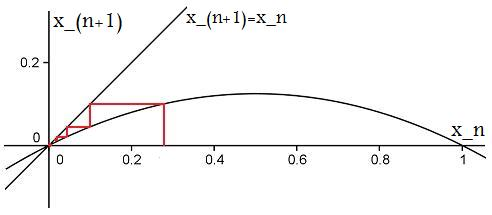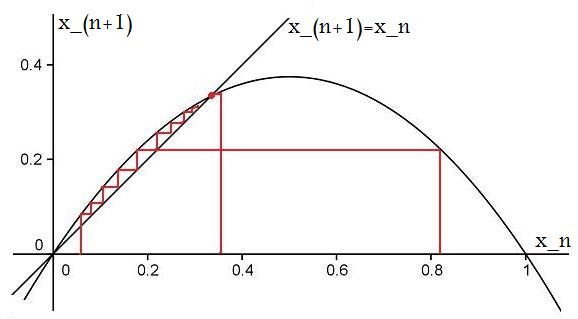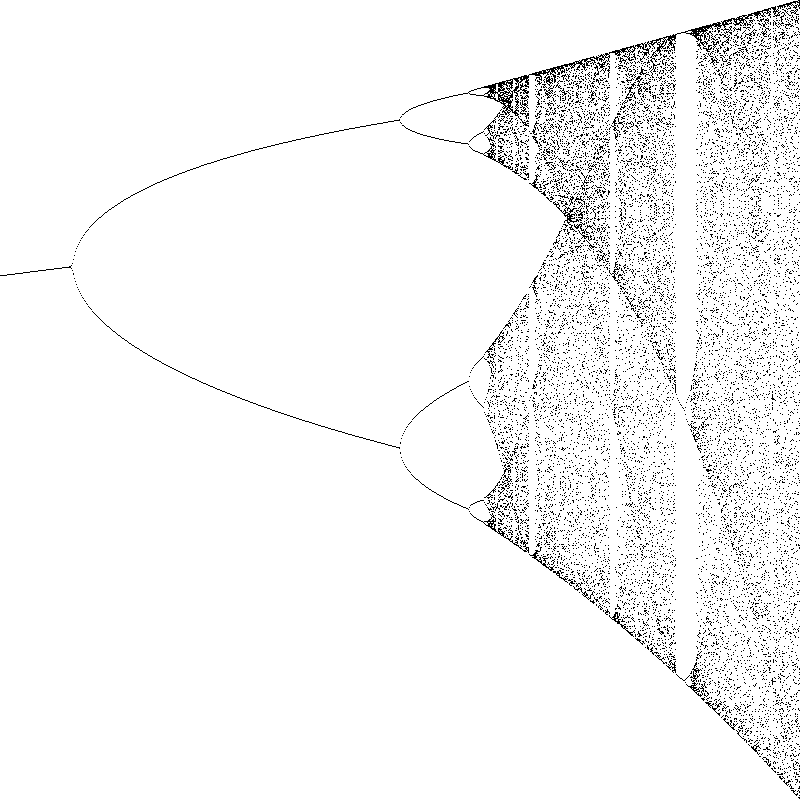#### You may also like### Ball Bearings

If a is the radius of the axle, b the radius of each ball-bearing, and c the radius of the hub, why does the number of ball bearings n determine the ratio c/a? Find a formula for c/a in terms of n.### Overarch 2

Bricks are 20cm long and 10cm high. How high could an arch be built without mortar on a flat horizontal surface, to overhang by 1 metre? How big an overhang is it possible to make like this?### Cushion Ball

The shortest path between any two points on a snooker table is the straight line between them but what if the ball must bounce off one wall, or 2 walls, or 3 walls?

# Population Dynamics - Part 4

### The Logistic Map

The logistic map is the discrete case of the logistic equation, given by:   $\frac {\mathrm{d}y}{\mathrm{d}t}=ry(1-\frac{y}{Y})$

We then approximate to deduce the discrete case:\begin{align*} \frac{y_{n+1}-y_n}{\Delta t} &\approx ry_n\left(1-\frac{y_n}{Y}\right) \\ y_{n+1} &\approx r \Delta t y_n \left(1-\frac{y_n}{Y}\right)+y_n \\ y_{n+1}&=(1+r \Delta t)y_n-\frac {r\Delta t}{Y}{(y_n)}^2 \\ y_{n+1}&=(1+r \Delta t)y_n\Bigg( 1-\bigg(\frac{r\Delta t}{1+r\Delta t}\bigg)\frac{y_n}{Y}\Bigg) \end{align*}

Let $\lambda=1+r\Delta t$ and $x_n=\frac {r\Delta t}{1+r \Delta t} \frac {y_n}{Y}$ . Then our equation becomes: $$x_{n+1}=\lambda x_n (1-x_n)$$ This is the logistic map. We can also think of it as a function $x_{n+1}=f(x_n)$.

### Finding Equilibrium Points

Question:   A fixed point implies $x_{n+1}=x_n$ . Find the fixed points by solving $$\lambda x_n (1-x_n) = x_n$$ To determine the stability of these points, we are going to find the stability, by investigating the function for values nearby the equilibrium points.

Start by supposing that $x_n=X$ is a fixed point. This means that $f(X)=X$.

To find a value near the equilibrium point, let $x_n=X+\epsilon{_n}$ where $\epsilon_n < < 1$. Then using the Taylor expansion: \begin{align*} x_{n+1}&=f(x_n) \\ X+\epsilon_{n+1} &= f(X+\epsilon_n) \\ &=f(X)+\epsilon_n f'(X)+... \end{align*}

We neglect the higher-order terms to get: $$X+\epsilon_{n+1}=f(X)+\epsilon_n f'(X)$$ Now from above we saw that $f(X)=X$ , so we can simplify to get: $$\epsilon_{n+1} \approx f'(X) \epsilon_n$$ A fixed point, X, is then stable if:   $\Bigg|\frac{\epsilon_{n+1}}{\epsilon_n}\Bigg | =\Bigg |f'(X)\Bigg | < 1$

Question:  Given that $f'(x)=\lambda-2\lambda x$ , find the stability of the fixed points $x_n=0$  and  $x_n=1-\frac{1}{\lambda}$

### Different Cases of Stability

Below are some graphs of the logistic map for different values of $\lambda$ .

Case 1: $\lambda< 1$

Only fixed point is 0, which is stable:Case 2: $1< \lambda < 2$

Unstable fixed point at 0 and stable fixed point at $1-\frac{1}{\lambda}$Question:  Can you find the stability for the case $2< \lambda < 3$  ?

Below is a picture of some fantastic fractal behaviour which occurs for $3< \lambda< 4$.Question:  Can you relate these values of $\lambda$ to what would actually be occuring in a population of organisms?